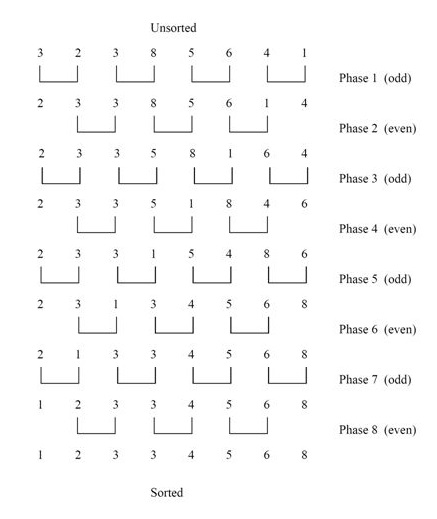# Odd-Even Sort Basic Information

suggest change

An Odd-Even Sort or brick sort is a simple sorting algorithm, which is developed for use on parallel processors with local interconnection. It works by comparing all odd/even indexed pairs of adjacent elements in the list and, if a pair is in the wrong order the elements are switched. The next step repeats this for even/odd indexed pairs. Then it alternates between odd/even and even/odd steps until the list is sorted.

Pseudo code for Odd-Even Sort:

``````if n>2 then
1. apply odd-even merge(n/2) recursively to the even subsequence a0, a2, ..., an-2 and to the odd subsequence a1, a3, , ..., an-1
2. comparison [i : i+1] for all i element {1, 3, 5, 7, ..., n-3}
else
comparison [0 : 1]``````

Wikipedia has best illustration of Odd-Even sort:Example of Odd-Even Sort:Implementation:

I used C# language to implement Odd-Even Sort Algorithm.

``````public class OddEvenSort
{
private static void SortOddEven(int[] input, int n)
{
var sort = false;

while (!sort)
{
sort = true;
for (var i = 1; i < n - 1; i += 2)
{
if (input[i] <= input[i + 1]) continue;
var temp = input[i];
input[i] = input[i + 1];
input[i + 1] = temp;
sort = false;
}
for (var i = 0; i < n - 1; i += 2)
{
if (input[i] <= input[i + 1]) continue;
var temp = input[i];
input[i] = input[i + 1];
input[i + 1] = temp;
sort = false;
}
}
}

public static int[] Main(int[] input)
{
SortOddEven(input, input.Length);
return input;
}
}``````

Auxiliary Space: `O(n)`

Time Complexity: `O(n)`